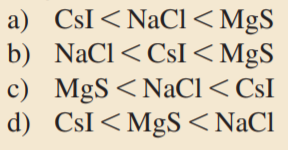×
Get Full Access to Chemistry: A Molecular Approach - 3 Edition - Chapter 9 - Problem 6saq
Get Full Access to Chemistry: A Molecular Approach - 3 Edition - Chapter 9 - Problem 6saq

×

# Which set of compounds is arranged in order of increasingISBN: 9780321809247 1

## Solution for problem 6SAQ Chapter 9

Chemistry: A Molecular Approach | 3rd Edition

• Textbook Solutions
• 2901 Step-by-step solutions solved by professors and subject experts
• Get 24/7 help from StudySoup virtual teaching assistantsChemistry: A Molecular Approach | 3rd Edition

4 5 1 309 Reviews
20
5
Problem 6SAQ

Which set of compounds is arranged in order of increasing magnitude of lattice energy?Step-by-Step Solution:

Solution 6SAQ Step 1 of 2 The lattice energy is the energy associated with the formation of a crystalline lattice of alternating cations and anions from the gaseous ions. Its value depends by ion dimensions because two ions cannot stay to close one to each other without raising the lattice energy. Lattice energies become less exothermic (less negative) with increasing ionic radius. Lattice energies become more exothermic (more negative) with increasing magnitude of ionic charge We know that ionic radii increases as we move down to a column and decreases as we move from the left to the right along a period. We are going to demonstrate that the correct answer is for letter a)

Step 2 of 2

##### ISBN: 9780321809247

Since the solution to 6SAQ from 9 chapter was answered, more than 520 students have viewed the full step-by-step answer. This full solution covers the following key subjects: arranged, compounds, Energy, increasing, lattice. This expansive textbook survival guide covers 82 chapters, and 9454 solutions. The answer to “?Which set of compounds is arranged in order of increasing magnitude of lattice energy?” is broken down into a number of easy to follow steps, and 14 words. Chemistry: A Molecular Approach was written by and is associated to the ISBN: 9780321809247. The full step-by-step solution to problem: 6SAQ from chapter: 9 was answered by , our top Chemistry solution expert on 02/22/17, 04:35PM. This textbook survival guide was created for the textbook: Chemistry: A Molecular Approach, edition: 3.

## Discover and learn what students are asking

Calculus: Early Transcendental Functions : Preparation for Calculus
?In Exercises 1–4, find any intercepts. $$y=\frac{x-3}{x-4}$$

Calculus: Early Transcendental Functions : Functions of Several Variables
?In Exercises 15 - 22, find all first partial derivatives. $$f(x, y)=4 x^{2}-2 x y+y^{2}$$

Statistics: Informed Decisions Using Data : Estimating a Population Proportion
?A ____________ is the value of a statistic that estimates the value of a parameter.

Unlock Textbook Solution

Enter your email below to unlock your verified solution to:

Which set of compounds is arranged in order of increasing